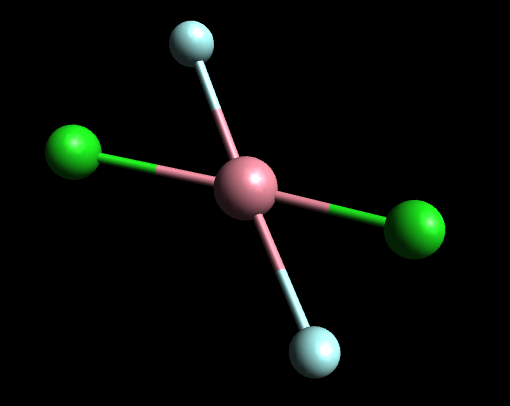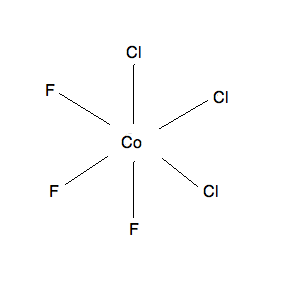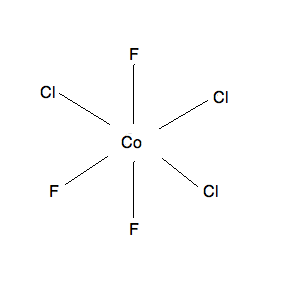# Stereoisomers: Geometric Isomers in Transition Metal Complexes II

Geometric Isomers are isomers that differ in the arrangement of the ligands around the metal or the central atom. In other words, these isomers differ from each other based on where the ligands are placed in the coordinate compound. This will be much easier to understand as examples will be considered. There are 2 main types of geometric isomers:

• Cis-Trans Isomers (referencing the spatial arrangement of two species)
• Mer-Fac Isomers (referencing the spatial arrangement of three species)

## Cis-Trans Isomers

Cis-Trans Isomers are isomers that differ in the arrangement of two ligands in square planar and octahedral geometry. Cis isomers are isomers where the two ligands are 90 degrees apart from one another in relation to the central molecule. This is because Cis isomers have a bond angle of 90o, between two same atoms. Trans isomers, on the other hand, are isomers where the two ligands are on opposite sides in a molecule because trans isomers have a bond angle of 180o, between the two same atoms. When naming cis or trans isomers, the name begins either with cis or trans, whichever applies, followed by a hyphen and then the name of a molecule. For example a cis isomer of CoCl2F2 would be called cis-CoCl2F2. Finally, the last thing to keep in mind when examining cis and trans isomers is that only square planar and octahedral geometries can have cis or trans isomers. Examples of both isomers are provided below.

Example $$\PageIndex{1}$$: cis Isomer

CoCl2F2:

(Color scheme: pink=cobalt, blue=fluorine, green=chlorine)This above is an example of the molecule cis-CoCl2F2 or cis-dichlorodifluorocobalt (IV). The molecule pictured above is a cis isomer because both fluorine and chlorine ligands, respectively, are on the same side of the molecule. Additionally, one can approximate that the bond angle between each of the chlorine atoms and between each of the fluorine atoms is 90o.

Isomer

CoCl2F2:

(Color scheme: pink=cobalt, blue=fluorine, green=chlorine)

(Note, the differences in the length of the bond between the two pictures are not intentional and have nothing to do with cis-trans isomerism)This above is an example of the molecule trans-CoCl2F2 or trans-dichlorodifluorocobalt (IV).

We know the above molecule is a trans isomer because the two same chlorine atoms and the two same fluorine atoms are opposite each other. Furthermore, the bond angle between the two chlorine atoms and between the two fluorine atoms is 180o. The above examples were all for square planar geometry but as the examples below illustrate, cis-trans isomerism can also occur in octahedral geometry. Both the molecules below are isomers of the molecule SCl2F4 (color scheme: yellow=sulfur, blue=fluorine, green=chlorine).

Example $$\PageIndex{3}$$: Octahedral cis Isomer

SCl2F4:We know this isomer above is a cis isomer because both the chlorine ligands are on the same side and the bond angle between the chlorine atoms appears to be 90o.

Example $$\PageIndex{4}$$: Octahedral trans Isomer

SCl2F4:The isomer above is a trans isomer because the chlorine ligands are on opposite sides and the bond angle between the chlorine atoms is 180o. All other isomers are essentially just rotations of these two isomers. Once again when trying to find cis and trans isomers look at the arrangement of the ligands. If two same ligands are on the same side, it is a cis isomer and if the ligands are on opposite sides, it is a trans isomer. Another way to tell the isomers apart is the bond angles: cis isomers have a 90o bond angle whereas trans isomers have a 180o bond angle.

## Mer-Fac Isomers

Mer-Fac isomers are easier to notice than cis-trans isomers in the sense that they only exist in octahedral geometry. Just like cis-trans isomers, mer-fac isomers are determined based on whether or not the ligands exist on the same side. Instead of dealing with 2 ligands, mer-fac isomers deal with 3 ligands. If the 3 ligands are all on the same side, the isomer is called a fac-isomer. Another way to identify fac isomers is to look at the bond angle between the ligands because fac isomers have a 90o bond angle between each of the 3 atoms. The mer isomer on the other hand is where only 2 of the 3 ligands are on the same side. In mer isomers, there exists a 90o-90o-180o bond angle between the 3 same ligands. In terms of nomenclature, mer-fac isomers follow the same rule as cis-trans isomers where you put the isomer type, followed by a hyphen, followed by the molecular formula. Examples have been provided below.

Example $$\PageIndex{5}$$: fac Isomer

Below is an example of the fac isomer, fac-CoCl3F3:(note the color scheme: pink=cobalt, green=chlorine, blue=fluorine)

Through the 2d version, it is easier to see how the ligands are all on the same side. Nonetheless, in the 3D version, one can observe that the bond angle between the 3 same ligands is 90o, thus making this isomer a fac-isomer.

Example $$\PageIndex{6}$$: mer Isomer

Below is an example of the mer isomer, mer-CoCl3F3:(note the color scheme: pink=cobalt, green=chlorine, blue=fluorine)

Its hard to tell in the 3D version but in the 2D version, one can easily tell how the same ligands are not on the same side. Additionally, one can approximate that the bond angle between the three chlorine atoms and between the three fluorine atoms is 90o-90o-180o, thus making the above molecule a mer isomer.